Remaining Power Rules

 Site: TBAISD Moodle Course: Michigan Algebra I Preview 2012 Book: Remaining Power Rules Printed by: Guest user Date: Sunday, January 17, 2021, 02:40 PM

Power of a Power

To simplify an expression that has an exponent raised to a power, multiply the existing exponent by the power.

Power of a Power Rule : (xn)m = xn·m

To see why this rule works, see the expansion below: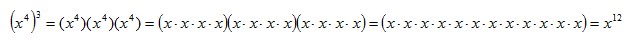Therefore,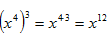.

Example

Simplify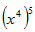.

Use the Power of a Power Rule to multiply the exponents.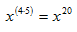Video Lesson

To see a video lesson about how to raise a power to a power, select the following link:

Power of a Product

When a product is inside the parentheses and a power is on the outside of the parentheses, then the outside power multiplies the exponent of each element inside.

Power of a Product Rule: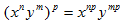*Note: This rule applies to a product only. If there is a sum or difference inside, refer to the steps of multiplying binomials.

Example 1 Simplify the following expression: (x2y)4

Step 1. Rewrite the expression with each element inside the parentheses to the power on the outside.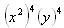Step 2. Use the Power of a Power Rule.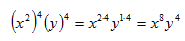Example 2 Simplify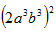.

Use the Power of a Product Rule to simplify the expression.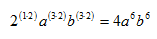Listen

Example 3

Simplify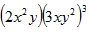.

Step 1. Use the Power of a Product Rule to simplify the expression.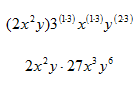Step 2. Use the Product of a Power Rule to simplify the expression.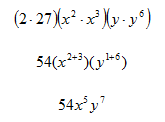Listen

Video Lesson

To see a video lesson about how to raise a product to a power, select the following link:

Guided Practice

To solidify your understanding of power of a product, visit the following link to Holt, Rinehart and Winston Homework Help Online. It provides examples, video tutorials and interactive practice with answers available. The Practice and Problem Solving section has two parts. The first part offers practice with a complete video explanation for the type of problem with just a click of the video icon. The second part offers practice with the solution for each problem only a click of the light bulb away.

Practice

Power of a Product Worksheet

Power of a Product Answer Key

Power of a Quotient

When the elements of a quotient have exponents and the quotient is raised to a power, then the power multiplies the exponent of each element in the quotient.

Power of a Quotient Rule :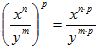.

Example 1

Simplify the expression: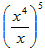.

Step 1. Use the Power of a Quotient Rule.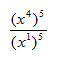Step 2. Use the Power of a Power Rule to simplify the resulting expression.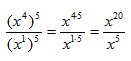Step 3. If the bases are the same, use the Quotient of a Power Rule for Exponents to simplify the resulting expression.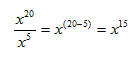Listen

Example 2

Simplify the expression: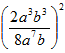.

Once familiar with the rules, the order of use will become apparent when simplifying expressions.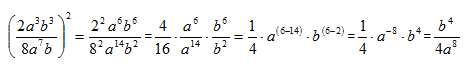Video Lesson

To see a video about how to raise a quotient to a power, select the following link:

Guided Practice

To solidify your understanding of raising a quotient to a power, visit the following link to Holt, Rinehart and Winston Homework Help Online. It provides examples, video tutorials and interactive practice with answers available. The Practice and Problem Solving section has two parts. The first part offers practice with a complete video explanation for the type of problem with just a click of the video icon. The second part offers practice with the solution for each problem only a click of the light bulb away.

Practice

Power of a Quotient Worksheet